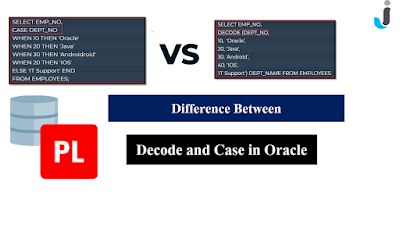### Ticker

6/recent/ticker-posts

# Difference Between Case and Decode in Oracle - Javainhand Tutorial

In this SQL Tutorial, We will explore about the Difference between Case and Decode in Oracle.In this blog post, we will understand some basic difference between Case and Decode in Oracle with the help of some questions and answer.

### Question 1:- What is Decode in Oracle?

Decode is a function in Oracle which helps to transfer your data to the another data. Decode helps to perform IF-THEN-ELSE logic in the SQL query.

### Question 2:- Syntax of the Decode Function

DECODE (expression, search, result [, search, result] ... [, default])

### Question 3:- Example of Decode Function in Oracle

```SELECT EMP_NO,
DECODE (DEPT_NO,
10,  'Oracle',
20, 'Java',
30, 'Android',
40, 'IOS',
'IT Support') DEPT_NAME FROM EMPLOYEES```

In the above example dept_no is expression. 10 is search value and Oracle is result.Let's understand in details if dept_no is 10 then decode function return Oracle, If dept_no is 20 then decode function return java same as for all and last is default if 10,20,30,40 dept_no is not found then decode function return TI support.

May be, Now above questions with answer will help you out for the better understanding about the Decode Function.

Now let's take some questions with answer on the Case Statement in Oracle.

### Question 1:- What is Case in Oracle?

Case is a statement in Oracle. It is also perform transfer your data to the another data. It is same as decode for perform IF-THEN-ELSE logic.

Case was introduced in Oracle 8.1. We can say it is an extended version of Decode.

### Question 2:- Syntax of Case Statement

CASE [expression]

WHEN condition_1 THEN result_1

WHEN condition_2 THEN result_2

ELSE result

WHEN condition_n THEN result_n

END case_name

### Question 3:- Example of Case Statement

```SELECT EMP_NO,
CASE DEPT_NO
WHEN 10 THEN 'Oracle'
WHEN 20 THEN 'Java'
WHEN 30 THEN 'Androidroid'
WHEN 20 THEN 'IOS'
ELSE 'IT Support' END
FROM EMPLOYEES;```

We are taking same example for the simple understanding. Now most the people ask me then what will we use for Decode or Case. So I have also explained the difference between Case and Decode in Oracle. The below comparison will help you out which is good for use.

## Difference Between CASE and DECODE in Oracle

In this section, you can easily understand the basic difference between CASE and DECODE. Why will I tell you that case is an extended version of DECODE.

### CASE

DECODE is a function.
CASE is a statement.
DECODE is not used in the WHERE clause.
CASE is used in the WHERE clause.
DECODE can be used only in SQL.
CASE can be used both SQL and PL/SQL
DECODE works with equality check(=)
CASE works with other relational operators like (>, <,>=, <=) as well equality check (=).
DECODE works with different data types.
CASE does not work with different data types.
DECODE does not pass as a parameter, Because DECODE only works with SQL Statements.
CASE does work as a parameter.

That's all for today I think (Difference Between Case and Decode in Oracle) post will helpful for you. If you like this post then share your view in the comment box.

### 2 Comments

1.1.If you have any doubts, Please let me know